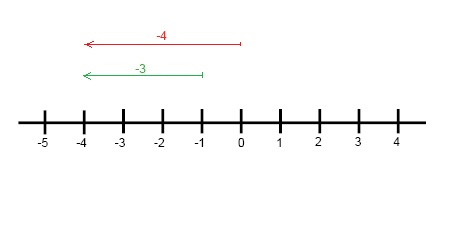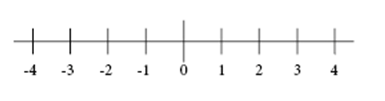# Absolute Value of an Integer

Absolute Value

In this section, you'll learn how to find the absolute value of integers.

Observe the following expressions carefully.

4 - 0 = 4
4 - 1 = 3
4 - 2 = 2
4 - 3 = 1
4 - 4 = 0
4 - 5 = -1

If you observed carefully, you can see that 4 - 5 is equal to a negative number i.e. -1, which is less than zero. A negative number is always less than zero, 0.

We can study this in a diagram by using two examples:  0 - 4 = -4 and -1 - 3 = -4The expressions above are shown on a number line. If you see, zero is always in the middle and separates negative and positive numbers on the left side and right side respectively. On the left side of zero, you'll find numbers that are less than zero, the negative numbers. On the right side of zero, you'll find numbers that are greater than zero, the positive numbers. The absolute value is the same as the distance from zero of a specific number.On the number line above, you can see that 4 and -4 are on the opposite sides of zero and they are the same distance from zero in opposite directions. They have the same absolute value, in this case 4.

Absolute value of an integer is its numerical value without taking the sign into consideration.

The absolute values of -9 = 9; the absolute value of 5 = 5 and so on.

The symbol used to denote the absolute value is, two vertical lines (| |), one on either side of an integer.

Therefore, if 'a' represents an integer, its absolute value is represented by |a| and is always non-negative.

Note:

(i) |a| = a; when 'a' is positive or zero.

(ii) |a| = -a; when 'a' is negative.

Examples on absolute value of an integer:

(i) Absolute value of - 7 is written as |- 7| = 7 [here mod of - 7 = 7]

(ii) Absolute value of + 2 is written as |+ 2| = 2 [here mod of + 2 = 2]

(iii) Absolute value of - 15 is written as |- 15| = 15 [here mod of - 15 = 15]

(iv) Absolute value of + 17 is written as |+ 17| = 17 [here mod of + 17 = 17]

Hope you understood Absolute Value of Integers. For more details visit - www.edusaksham.com